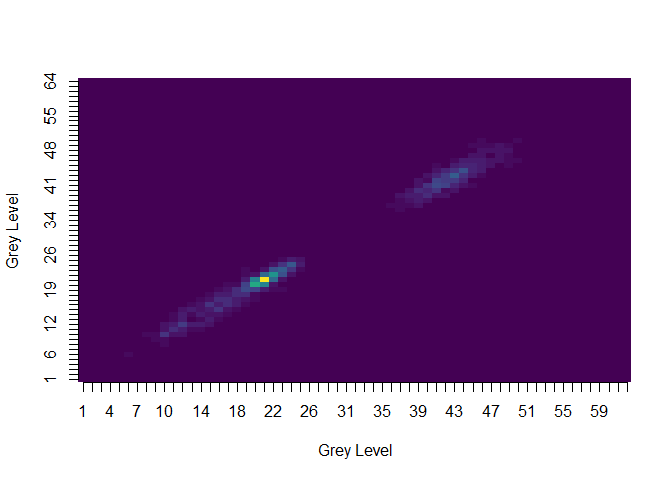### ** Not Currently Maintained **

##### This project is not currently being maintained. While I will do my best to help in a timely fashion, you should not expect a prompt response.

The `radiomics` package is a set of tools for computing texture matrices and features from images.

The release version of this package (April 2016, v0.1.2) is available from CRAN using:

``````install.packages("radiomics")
``````

Or you can install the development version of the package using:

``````devtools::install_github("joelcarlson/radiomics")

``````

# Texture Matrices

In the package are functions for calculating four different types of matrices and associated feature sets used to quantify the texture of an image.

These matrices are the:

• Grey Level Co-occurrence Matrix
• Grey Level Run Length Matrix
• Grey Level Size Zone Matrix
• Multiple Grey Level Size Zone Matrix

Detailed usage directions for calculating features and matrices can be found in the package vignette (use ```browseVignettes(package = "radiomics")```)

# Using the Package

## Building Texture Matrices

Texture matrices can be created from 2D images by using the abbreviated and lowercase matrix name as a function call:

``````tumor <- radiomics::tumor #2D MRI slice of a brain tumor
glcm(tumor)
glrlm(tumor)
glszm(tumor)
mglszm(tumor)
``````

A matrix with the class of the texture matrix type is returned, as shown here using `glcm(tumor, n_grey=4)`

``````#> An object of class "glcm"
#>              1          2           3            4
#> 1 0.1617021277 0.03356974 0.001891253 0.0004728132
#> 2 0.0335697400 0.38345154 0.010638298 0.0014184397
#> 3 0.0018912530 0.01063830 0.301654846 0.0184397163
#> 4 0.0004728132 0.00141844 0.018439716 0.0203309693
``````
``````class(glcm(tumor, n_grey=4))
#>  "glcm"
``````

## Visualizing Texture Matrices

Each matrix type has an associated `image` function for visualization of the results:

``````image(glcm(tumor))
image(glrlm(tumor))
image(glszm(tumor))
image(mglszm(tumor))
``````

The `image` functions make use of the `viridis` scale, as shown here using `image(glcm(tumor, n_grey=64))`:## Calculating Features

Each matrix type has an associated `calc_features` function, which returns an object of class `data.frame` with a single observation for each calculated feature. First order features can also be calculated on 2D matrices.

``````calc_features(tumor)
calc_features(glcm(tumor))
calc_features(glrlm(tumor))
calc_features(glszm(tumor))
calc_features(mglszm(tumor))
``````